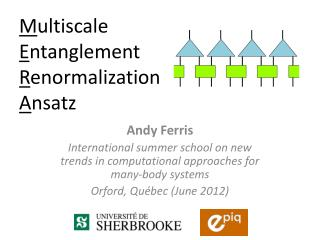# M ultiscale E ntanglement R enormalization A nsatz - PowerPoint PPT PresentationDownload PresentationM ultiscale E ntanglement R enormalization A nsatz

M ultiscale E ntanglement R enormalization A nsatz
Download Presentation## M ultiscale E ntanglement R enormalization A nsatz

- - - - - - - - - - - - - - - - - - - - - - - - - - - E N D - - - - - - - - - - - - - - - - - - - - - - - - - - -
##### Presentation Transcript

1. MultiscaleEntanglementRenormalizationAnsatz Andy Ferris International summer school onnew trends in computational approaches for many-body systems Orford, Québec (June 2012)

2. What will I talk about? • Part one (this morning) • Entanglement and correlations in many-body systems • MERA algorithms • Part two (this afternoon) • 2D quantum systems • Monte Carlo sampling • Future directions…

3. Outline: Part 1 • Entanglement, critical points, scale invariance • Renormalization group and disentangling • The MERA wavefunction • Algorithms for the MERA • Extracting expectation values • Optimizing ground state wavefunctions • Extracting scaling exponents (conformal data)

4. Entanglement in many-body systems • A general, entangled state requires exponentially many parameters to describe (in number of particles N or system size L) • However, most states of interest (e.g. ground states, etc) have MUCH less entanglement. • Explains success of many variational methods • DMRG/MPS for 1D systems • and PEPS for 2D systems • and now, MERA

5. Boundary or Area law for entanglement 1D: 2D: 3D: =

6. Obeying the area law: 1D gapped systems • All gapped 1D systems have bounded entanglement in ground state (Hastings, 2007) • Exists an MPS that is a good approximation

7. Violating the area law: free fermions • However, simple systems can violate area law , for an MPS we need Energy Fermi level Momentum

8. Critical points Wikipedia ltl.tkk.fi Low Temperature Lab, Aalto University Simon et al., Nature472, 307–312 (21 April 2011)

9. Violating the area law: critical systems • Correlation length diverges when approaching critical point • Naïve argument for area law (short range entanglement) fails. • Usually, we observe a logarithmic violation: • Again, MPS/DMRG might become challenging.

10. Scale-invariance at criticality • Near a (quantum) critical point, (quantum) fluctuations appear on all length scales. • Remember: quantum fluctuation = entanglement • On all length scales implies scale invariance. • Scale invariance implies polynomially decaying correlations • Critical exponents depend on universality class

11. MPS have exponentially decaying correlations Take a correlator:

12. MPS have exponentially decaying correlations Take a correlator:

13. MPS have exponentially decaying correlations Exponential decay:

14. Renormalization group • In general, the idea is to combine two parts (“blocks”) of a systems into a single block, and simplify. • Perform this successively until there is a simple, effective “block” for the entire system. =

15. Momentum-space renormalization Numerical renormalization group (Wilson) Kondo: couple impurity spin to free electrons Idea: Deal with low momentum electrons first

16. Real-space renormalization = = = =

17. Tree tensor network as a unitary quantum circuit Every tree can be written with isometric/unitary tensors with QR decomposition

18. Tree tensor network as a unitary quantum circuit Every tree can be written with isometric/unitary tensors with QR decomposition

19. Tree tensor network as a unitary quantum circuit Every tree can be written with isometric/unitary tensors with QR decomposition

20. Tree tensor network as a unitary quantum circuit Every tree can be written with isometric/unitary tensors with QR decomposition

21. The problem with trees:short range entanglement MPS-like entanglement! =

22. Idea: remove the short range entanglement first! • For scale-invariant systems, short-range entanglement exists on all length scales • Vidal’s solution: disentangle the short-range entanglement before each coarse-graining Local unitary to remove short-range entanglement

23. New ansatz: MERA Each Layer : Coarse-graining Disentangle

24. New ansatz: MERA 2 sites 4 sites 8 sites 16 sites

25. Properties of the MERA • Efficient, exact contractions • Cost polynomial in , e.g. • Allows entanglement up to • Allows polynomially decaying correlations • Can deal with finite (open/periodic) systems or infinite systems • Scale invariant systems

26. Efficient computation: causal cones 2 sites 3 sites 3 sites 2 sites = =

27. Causal cone width • The width of the causal cone never grows greater than 3… • This makes all computations efficient!

28. Efficient computation: causal cones

29. Efficient computation: causal cones

30. Efficient computation: causal cones

31. Efficient computation: causal cones

32. Other MERA structures • MERA can be modified to fit boundary conditions • Periodic • Open • Finite-correlated • Scale-invariant • Also, renormalization scheme can be modified • E.g. 3-to-1 transformations = ternary MERA • Halve the number of disentanglers for efficiency

33. Periodic Boundaries

34. Open Boundaries

35. Finite-correlated MERA Good for non-critical systems Maximum length of correlations/entanglement

36. Scale-invariant MERA

37. Correlations in a scale-invariant MERA • “Distance” between points via the MERA graph is logarithmic • Some “transfer op-erator” is applied times. = =

38. MERA algorithms Certain tasks are required to make use of the MERA: • Expectation values • Equivalently, reduced density matrices • Optimizing the tensor network (to find ground state) • Applying the renormalization procedure • Transform to longer or shorter length scales

39. Local expectation values

40. Global expectation values This if fine, but sometimes we want to take the expecation value of something translationally invariant, say a nearest-neighbour Hamiltonian. We can do this with cost (or with constant cost for the infinite scale-invariant MERA).

41. A reduced density matrix

42. Solution: find reduced density matrix • We can find the reduced density matrix averaged over all sites • Realize the binary MERA repeats one of two structures at each layer, for 3-body operators

43. Reduced density matrix at each length scale